Дана: 0

Барлыгы: 0,00

0

# Regular square pyramid

### Regular square pyramid

A regular square pyramid is a right pyramid with a square base and four triangular faces.

Математика

Этикеткалар

математика, геометрия, solid geometry, solids, grouping of solids, pyramid, surface, көлем, definition, motherboard, lateral surface, face, height, formula, right pyramid, тетраэдр, regular solids, oblique pyramid, vertices, faces, edges

Байланысты экстралар

### Көріністер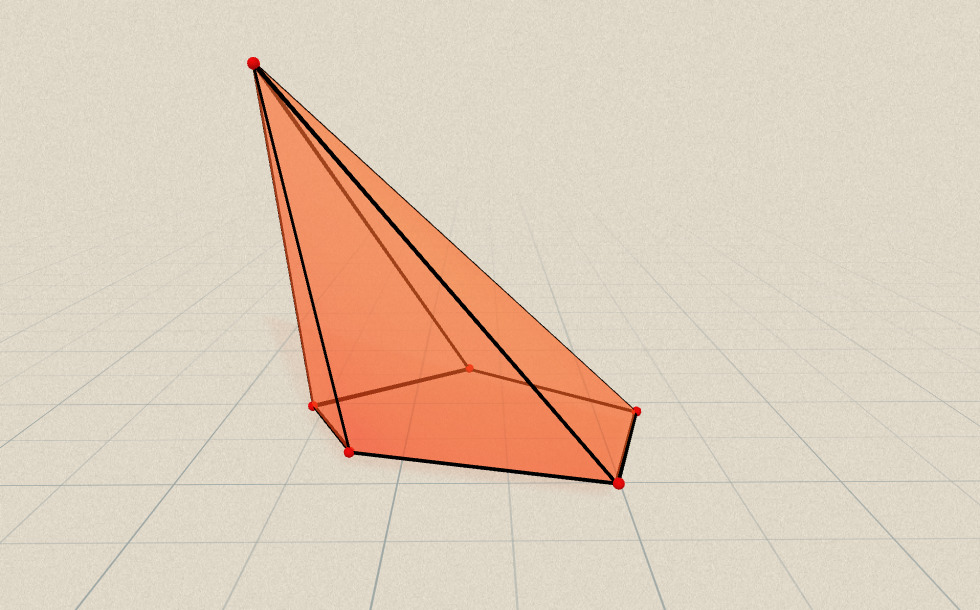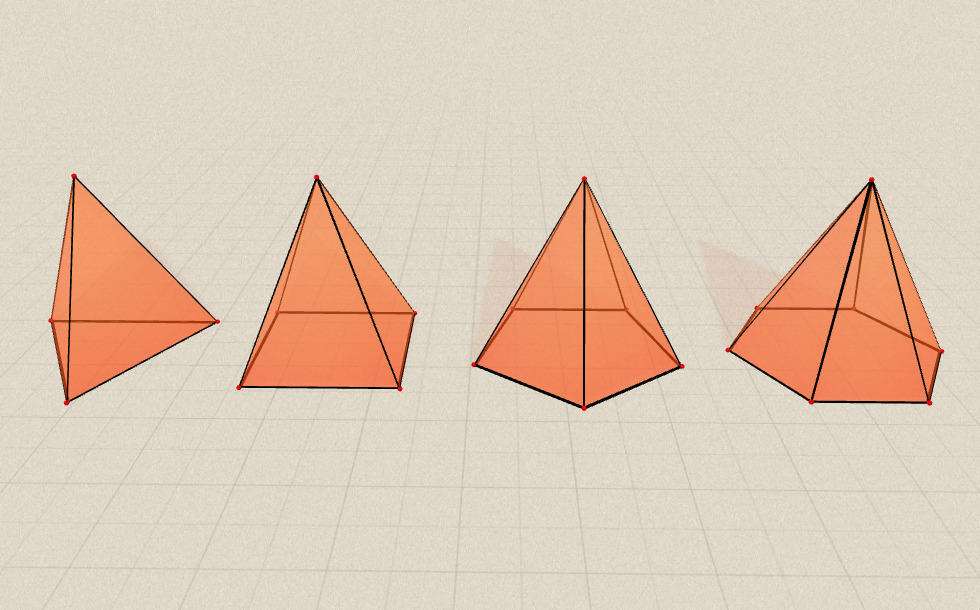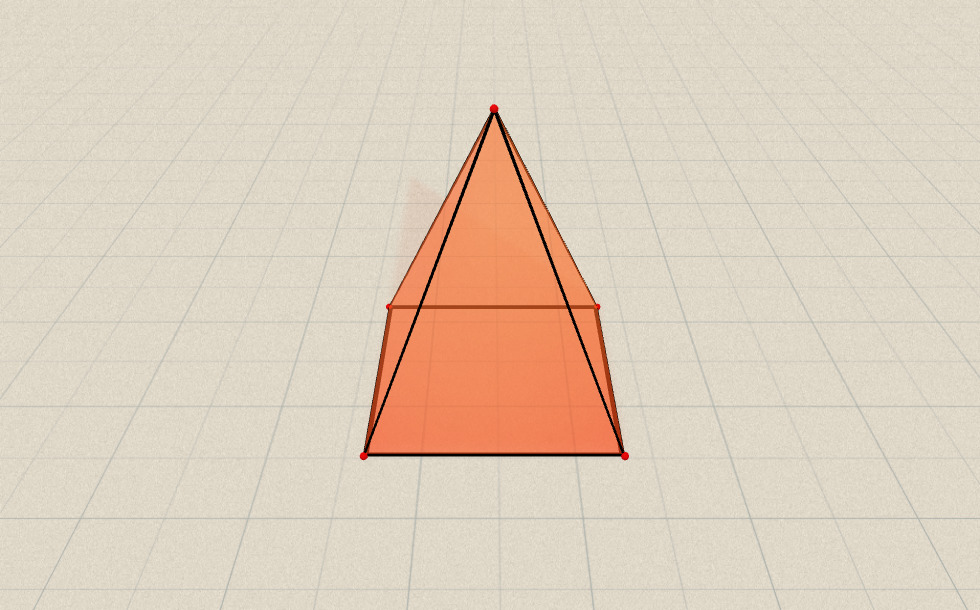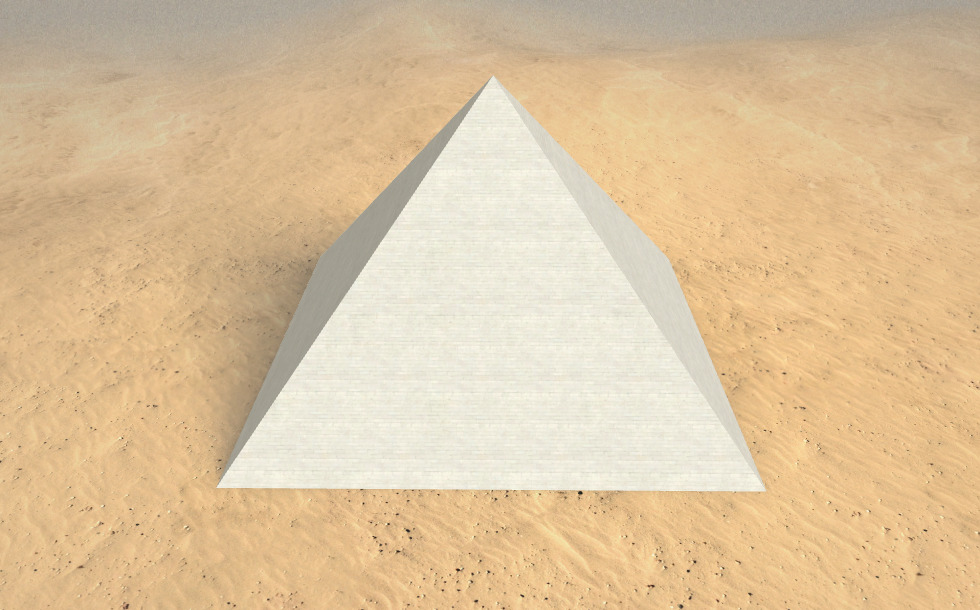### Байланысты экстралар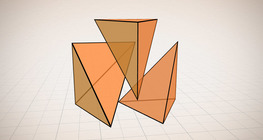#### Volume of a tetrahedron

To calculate the volume of a tetrahedron we start by calculating the volume of a prism.#### Жазық фигуралардың периметрі мен ауданын, беттің ауданын және көлемін есептеу

Анимацияның көмегімен жазық фигуралардың периметрі мен ауданын есептеу формуласымен,...#### Cube sections (exercise)

Examining solids formed by the intersection of a cube and a plane.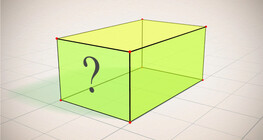#### Cuboid (exercises)

Edges, diagonals and faces of a cuboid can be identified by its vertices.#### Euler's polyhedron formula

The theorem formulated by Leonhard Euler describes one of the basic properties of convex...#### Grouping of solids 1

This animation demonstrates various groups of solids through examples.#### Grouping of solids 2

This animation demonstrates various groups of solids through examples.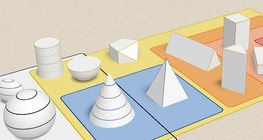#### Grouping of solids 3

This animation demonstrates various groups of solids through examples.#### Grouping of solids 4

This animation demonstrates various groups of solids through examples.#### Platonic solids

This animation demonstrates the five regular three-dimensional (or Platonic) solids, the...#### Ratio of volumes of similar solids

This 3D scene explains the correlation between the ratio of similarity and the ratio of...#### Solids of revolution

Rotating a geometric shape around a line within its geometric plane as an axis results in...#### Мысыр пирамидалары (Гиза, б.з.д. 26 ғасыр)

Гиза пирамидалары жеті ғажайыптың ішінде осы заманға дейін жеткен ерте дәуірдің...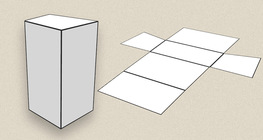#### Prisms

This animation demonstrates several types of prisms, from general to regular.